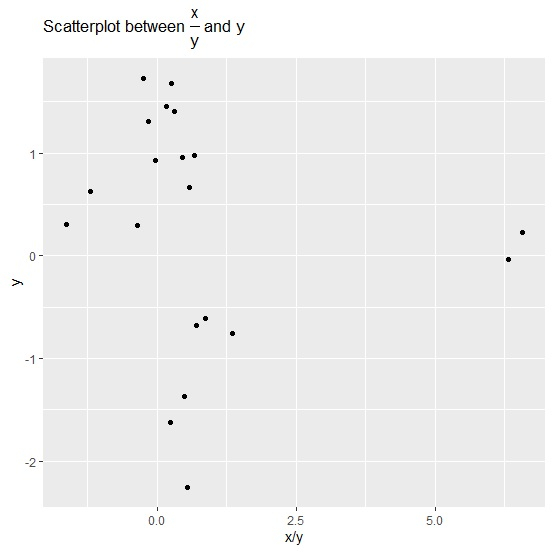# How to display fraction in a plot title using ggplot2 in R?

To display fraction in a plot title using ggplot2 in R, we can use frac function inside ggtitle function of ggplot2 package. Generally, fraction is displayed as X/Y but frac function helps to create the fraction in over form.

Check out the below example to understand how over form of fraction can be displayed in a plot title using ggplot2.

## Example

Following snippet creates a sample data frame −

x<-rnorm(20)
y<-rnorm(20)
df<-data.frame(x,y)
df

The following dataframe is created −

       x            y
1   0.23483738  1.4506593
2  -0.53391645 -0.6103884
3   0.43178470  0.9607968
4  -0.02202213  0.9308459
5  -0.67083342 -1.3704258
6  -1.01972845 -0.7536449
7  -0.41450037  1.7233977
8   0.65886251  0.9757482
9   1.47215186  0.2239160
10 -0.50323646  0.3078364
11 -0.75307929  0.6244623
12  0.43689883  1.4064075
13 -0.47781745 -0.6764910
14 -0.21645828  1.3042513
15 -0.39136288 -1.6218892
16 -0.10340456  0.2913214
17  0.41817242  1.6793330
18 -1.21634661 -2.2519936
19 -0.20690016 -0.0327693
20  0.38731829  0.6627035

To load ggplot2 package and create point chart between x and y with plot title displaying fraction in over form, add the following code to the above snippet −

library(ggplot2)
ggplot(df,aes(x/y,y))+geom_point()+ggtitle(expression(paste("Scatterplot between ", frac(x,y), " and y")))

## Output

If you execute all the above given snippets as a single program, it generates the following output −